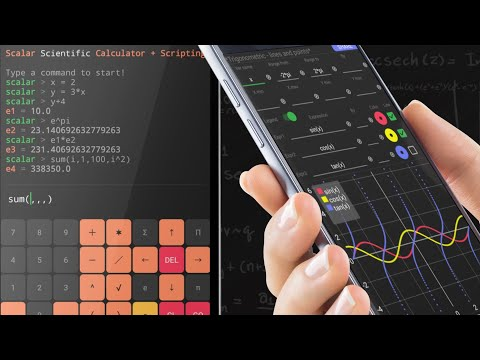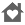Scalar Pro — Advanced Calculator & Math ScriptsEveryone
214⭐️⭐️⭐️⭐️⭐️ Before purchasing, you can try the free version: Scalar Lite

🥇 Extremely flexible function calculator and math scripting + beautiful design!

Scalar is much more than a function calculator. Scalar is a powerful math engine and math scripting language, that combines the simplicity of standard calculators with the flexibility of scripting. Thanks to Scalar, defining arguments and functions, as well as using them in subsequent calculations, expressions and functions graphs, has never been easier. You will see it shortly after getting familiar with available screens and options.

🎯

Main features:

🔹 Standard & Advanced Scientific Calculator
🔹 Extremely convenient calculator keyboard
🔹 Re-usage of previous calculations, just reference a constant that was created for your convenience
🔹 User defined arguments, as simple as x = 2
🔹 User defined functions, as simple as f(x) = x^2, f(x,y,…)=2*x+y
🔹 User defined random variables, as simple as rand X = rNor(0,1)+1
🔹 Beautiful function graphs, set variables, range, expression, interact with the chart!
🔹 Scripts writing, personalize and automate you work!
🔹 Rich set of examples built-in in the app!
🔹 Work saving and results sharing

The definition of user elements has never been easier!

Using Scalar you can easily create user elements, below are examples of the natural mathematical syntax:

scalar > x = 2
scalar > y = 2 * x
scalar > y
e1 = 4.0

scalar > x = 3
scalar > y
e2 = 6.0

There is no need to save the results!

In Scalar, each result is assigned to an automatically created constant, see example:

scalar > 2 + 3
e1 = 5.0

scalar > 4 + 6
e2 = 10.0

scalar > e1 + e2
✪ ➥ e3 = 15.0

User functions give huge possibilities of personalization!

Defining user functions is just as simple as writing a formula

scalar > f (x, y) = sqrt (x ^ 2 + y ^ 2)
scalar > f (3,4)
e1 = 5.0

👩‍🏫

Scalar was created by a mathematician, therefore it has built-in summation and product operators!

Scalar also supports summation and product operators, for example the number of prime numbers in the range of 2 to 1000

scalar > sum ( i, 2, 10000, ispr (i) )
e1 = 1229.0

⚡️

This is only a tiny part of available options!

It is only a presentation of a small part of the available mathematical functions. All implemented math elements exceeds several hundred.

👩🏻‍💻

At Scalar, you can write scripts!

🔹 The ability to write scripts is a unique feature among scientific calculators.
🔹 We all know how scripts significantly accelerate work.
🔹 Scalar provides a nice script editor with syntax highlighting & syntax hints.
🔹 Scripts can be saved and/or shared (pro version).
🔹 Start-up script is also supported (pro version).

📈

At Scalar you can create beautiful function graphs!

🔹 Visualization is important - no doubts!
🔹 Scalar provides the ability to create highly personalized function charts.
🔹 Functions graphs are fully interactive: values reading, scaling, zooming.
🔹 Function charts can be saved or shared (pro version).

📳

ScalarMath.org

More details on: ScalarMath.org

👌

Enjoy using Scalar!

Collapse

Review Policy
4.8
214 total
5
4
3
2
1

What's New

Collapse

Eligible for Family LibraryEligible if bought after 7/2/2016. Learn More
Updated
April 7, 2019
Size
8.5M
Installs
10,000+
Current Version
1.1.9
Requires Android
4.2 and up
Content Rating
Everyone
Permissions
Offered By
Scalar Math
Developer
Omulewska 9/24 04-128 Warszawa Poland